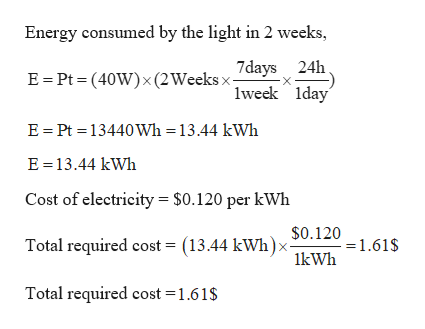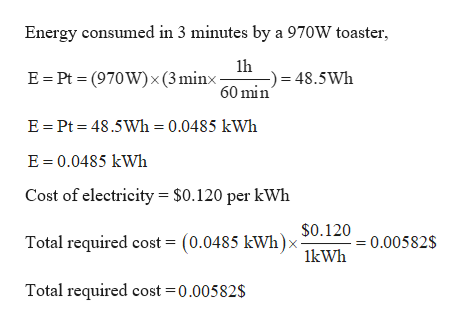# The cost of electricity varies widely throughout the UnitedStates; \$0.120/kWh is a typical value. At this unit price, calculatethe cost of (a) leaving a 40.0 - W porch light on for 2 weekswhile you are on vacation, (b) making a piece of dark toast in3.00 min with a 970 - W toaster, and (c) drying a load of clothesin 40.0 min in a 5 200 - W dryer.

Question
12 views

The cost of electricity varies widely throughout the United
States; \$0.120/kWh is a typical value. At this unit price, calculate
the cost of (a) leaving a 40.0 - W porch light on for 2 weeks
while you are on vacation, (b) making a piece of dark toast in
3.00 min with a 970 - W toaster, and (c) drying a load of clothes
in 40.0 min in a 5 200 - W dryer.

check_circle

Step 1

Given:

Cost of electricity = \$0.120 per kWh

Step 2

Calculating the cost of leaving a 40 W porch light on for 2 weeks:help_outlineImage TranscriptioncloseEnergy consumed by the light in 2 weeks, 7days 24h lweek Iday E = Pt = (40W)x(2Weeksx- E = Pt = 13440Wh =13.44 kWwh E =13.44 kWh Cost of electricity = \$0.120 per kWh \$0.120 :=1.61\$ Total required cost = (13.44 kWh)> 1kWh Total required cost =1.61\$ fullscreen
Step 3

Calculating the cost of making a piece of dark toast in 3.00&n...help_outlineImage TranscriptioncloseEnergy consumed in 3 minutes by a 970W toaster, 1h -) = 48.5Wh E = Pt = (970W)×(3minx- 60 min E= Pt = 48.5Wh = 0.0485 kWh E = 0.0485 kWh Cost of electricity = \$0.120 per kWh \$0.120 (0.0485 kWh)> = 0.00582\$ Total required cost = 1kWh Total required cost =0.00582\$ fullscreen

### Want to see the full answer?

See Solution

#### Want to see this answer and more?

Solutions are written by subject experts who are available 24/7. Questions are typically answered within 1 hour.*

See Solution
*Response times may vary by subject and question.
Tagged in

### Physics